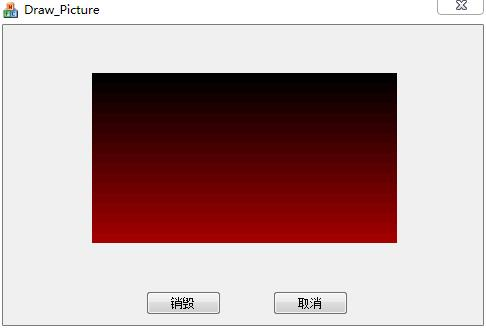MFC如何按一下按钮绘制图片，再按一下同一个按钮销毁图片？ 5C

MFC如何按一下按钮绘制图片，再按一下同一个按钮销毁图片？按钮响应事件里的代码应该如何去写？（有代码就好了）

1个回答

//声明一个全局变量
BOOL m_bIsDraw = TRUE;
//执行函数
void CDraw_PictureDlg::OnBnClickedOk()
{
if(m_bIsDraw == TRUE)
{
Draw_Pic();
m_bIsDraw = FALSE;
GetDlgItem(IDOK)->SetWindowText(_T("销毁"));
}
else
{
Destroy_Pic();
m_bIsDraw = TRUE;
GetDlgItem(IDOK)->SetWindowText(_T("绘制"));
}
}

void CDraw_PictureDlg::Draw_Pic()
{
CDC* pDC = m_Static_Pic.GetDC();
CRect rc;
m_Static_Pic.GetClientRect(&rc);

``````/*用渐变色粉刷背景*/
CRect rect(0,0,0,0);
for (int i = 0; i <= rc.Height(); i++)
{
rect.left = 0;
rect.right = rc.right;
rect.top = i * 10;
rect.bottom = (i + 1) * 10;
if(rect.bottom > rc.Height())
break;

pDC->FillSolidRect(&rect, RGB((i * 10) % 255, 0, 0));
}
``````

}

void CDraw_PictureDlg::Destroy_Pic()
{
m_Static_Pic.Invalidate();
}